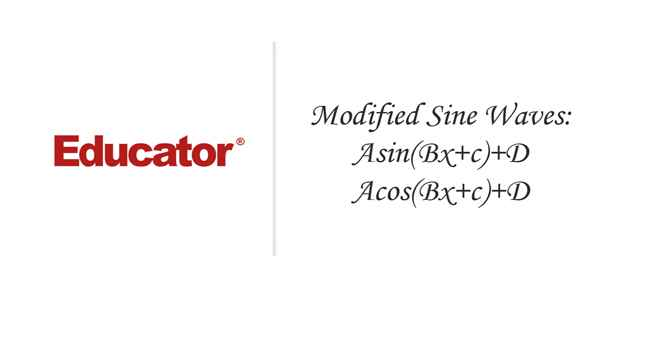Professor Murray

Modified Sine Waves: Asin(Bx+C)+D and Acos(Bx+C)+D

Slide Duration:

Section 1: Trigonometric Functions
Angles

39m 5s

Intro
0:00
Degrees
0:22
Circle is 360 Degrees
0:48
Splitting a Circle
1:13
2:08
2:31
2:52
Half-Circle and Right Angle
4:00
6:24
6:52
Coterminal, Complementary, Supplementary Angles
7:23
Coterminal Angles
7:30
Complementary Angles
9:40
Supplementary Angles
10:08
Example 1: Dividing a Circle
10:38
Example 2: Converting Between Degrees and Radians
11:56
Example 3: Quadrants and Coterminal Angles
14:18
Extra Example 1: Common Angle Conversions
-1
Extra Example 2: Quadrants and Coterminal Angles
-2
Sine and Cosine Functions

43m 16s

Intro
0:00
Sine and Cosine
0:15
Unit Circle
0:22
Coordinates on Unit Circle
1:03
Right Triangles
1:52
2:25
Master Right Triangle Formula: SOHCAHTOA
2:48
Odd Functions, Even Functions
4:40
Example: Odd Function
4:56
Example: Even Function
7:30
Example 1: Sine and Cosine
10:27
Example 2: Graphing Sine and Cosine Functions
14:39
Example 3: Right Triangle
21:40
Example 4: Odd, Even, or Neither
26:01
Extra Example 1: Right Triangle
-1
Extra Example 2: Graphing Sine and Cosine Functions
-2
Sine and Cosine Values of Special Angles

33m 5s

Intro
0:00
45-45-90 Triangle and 30-60-90 Triangle
0:08
45-45-90 Triangle
0:21
30-60-90 Triangle
2:06
Mnemonic: All Students Take Calculus (ASTC)
5:21
Using the Unit Circle
5:59
New Angles
6:21
9:43
Mnemonic: All Students Take Calculus
10:13
13:11
16:48
Example 3: All Angles and Quadrants
20:21
Extra Example 1: Convert, Quadrant, Sine/Cosine
-1
Extra Example 2: All Angles and Quadrants
-2
Modified Sine Waves: Asin(Bx+C)+D and Acos(Bx+C)+D

52m 3s

Intro
0:00
Amplitude and Period of a Sine Wave
0:38
Sine Wave Graph
0:58
Amplitude: Distance from Middle to Peak
1:18
Peak: Distance from Peak to Peak
2:41
Phase Shift and Vertical Shift
4:13
Phase Shift: Distance Shifted Horizontally
4:16
Vertical Shift: Distance Shifted Vertically
6:48
Example 1: Amplitude/Period/Phase and Vertical Shift
8:04
Example 2: Amplitude/Period/Phase and Vertical Shift
17:39
Example 3: Find Sine Wave Given Attributes
25:23
Extra Example 1: Amplitude/Period/Phase and Vertical Shift
-1
Extra Example 2: Find Cosine Wave Given Attributes
-2
Tangent and Cotangent Functions

36m 4s

Intro
0:00
Tangent and Cotangent Definitions
0:21
Tangent Definition
0:25
Cotangent Definition
0:47
Master Formula: SOHCAHTOA
1:01
Mnemonic
1:16
Tangent and Cotangent Values
2:29
Remember Common Values of Sine and Cosine
2:46
90 Degrees Undefined
4:36
Slope and Menmonic: ASTC
5:47
Uses of Tangent
5:54
Example: Tangent of Angle is Slope
6:09
7:49
Example 1: Graph Tangent and Cotangent Functions
10:42
Example 2: Tangent and Cotangent of Angles
16:09
Example 3: Odd, Even, or Neither
18:56
Extra Example 1: Tangent and Cotangent of Angles
-1
Extra Example 2: Tangent and Cotangent of Angles
-2
Secant and Cosecant Functions

27m 18s

Intro
0:00
Secant and Cosecant Definitions
0:17
Secant Definition
0:18
Cosecant Definition
0:33
Example 1: Graph Secant Function
0:48
Example 2: Values of Secant and Cosecant
6:49
Example 3: Odd, Even, or Neither
12:49
Extra Example 1: Graph of Cosecant Function
-1
Extra Example 2: Values of Secant and Cosecant
-2
Inverse Trigonometric Functions

32m 58s

Intro
0:00
Arcsine Function
0:24
Restrictions between -1 and 1
0:43
Arcsine Notation
1:26
Arccosine Function
3:07
Restrictions between -1 and 1
3:36
Cosine Notation
3:53
Arctangent Function
4:30
Between -Pi/2 and Pi/2
4:44
Tangent Notation
5:02
Example 1: Domain/Range/Graph of Arcsine
5:45
Example 2: Arcsin/Arccos/Arctan Values
10:46
Example 3: Domain/Range/Graph of Arctangent
17:14
Extra Example 1: Domain/Range/Graph of Arccosine
-1
Extra Example 2: Arcsin/Arccos/Arctan Values
-2
Computations of Inverse Trigonometric Functions

31m 8s

Intro
0:00
Inverse Trigonometric Function Domains and Ranges
0:31
Arcsine
0:41
Arccosine
1:14
Arctangent
1:41
Example 1: Arcsines of Common Values
2:44
Example 2: Odd, Even, or Neither
5:57
Example 3: Arccosines of Common Values
12:24
Extra Example 1: Arctangents of Common Values
-1
Extra Example 2: Arcsin/Arccos/Arctan Values
-2
Section 2: Trigonometric Identities
Pythagorean Identity

19m 11s

Intro
0:00
Pythagorean Identity
0:17
Pythagorean Triangle
0:27
Pythagorean Identity
0:45
Example 1: Use Pythagorean Theorem to Prove Pythagorean Identity
1:14
Example 2: Find Angle Given Cosine and Quadrant
4:18
Example 3: Verify Trigonometric Identity
8:00
Extra Example 1: Use Pythagorean Identity to Prove Pythagorean Theorem
-1
Extra Example 2: Find Angle Given Cosine and Quadrant
-2
Identity Tan(squared)x+1=Sec(squared)x

23m 16s

Intro
0:00
Main Formulas
0:19
Companion to Pythagorean Identity
0:27
For Cotangents and Cosecants
0:52
How to Remember
0:58
Example 1: Prove the Identity
1:40
Example 2: Given Tan Find Sec
3:42
Example 3: Prove the Identity
7:45
Extra Example 1: Prove the Identity
-1
Extra Example 2: Given Sec Find Tan
-2

52m 52s

Intro
0:00
0:09
How to Remember
0:48
Cofunction Identities
1:31
How to Remember Graphically
1:44
Where to Use Cofunction Identities
2:52
Example 1: Derive the Formula for cos(A-B)
3:08
Example 2: Use Addition and Subtraction Formulas
16:03
Example 3: Use Addition and Subtraction Formulas to Prove Identity
25:11
Extra Example 1: Use cos(A-B) and Cofunction Identities
-1
Extra Example 2: Convert to Radians and use Formulas
-2
Double Angle Formulas

29m 5s

Intro
0:00
Main Formula
0:07
How to Remember from Addition Formula
0:18
Two Other Forms
1:35
Example 1: Find Sine and Cosine of Angle using Double Angle
3:16
Example 2: Prove Trigonometric Identity using Double Angle
9:37
Example 3: Use Addition and Subtraction Formulas
12:38
Extra Example 1: Find Sine and Cosine of Angle using Double Angle
-1
Extra Example 2: Prove Trigonometric Identity using Double Angle
-2
Half-Angle Formulas

43m 55s

Intro
0:00
Main Formulas
0:09
Confusing Part
0:34
Example 1: Find Sine and Cosine of Angle using Half-Angle
0:54
Example 2: Prove Trigonometric Identity using Half-Angle
11:51
Example 3: Prove the Half-Angle Formula for Tangents
18:39
Extra Example 1: Find Sine and Cosine of Angle using Half-Angle
-1
Extra Example 2: Prove Trigonometric Identity using Half-Angle
-2
Section 3: Applications of Trigonometry
Trigonometry in Right Angles

25m 43s

Intro
0:00
Master Formula for Right Angles
0:11
SOHCAHTOA
0:15
Only for Right Triangles
1:26
Example 1: Find All Angles in a Triangle
2:19
Example 2: Find Lengths of All Sides of Triangle
7:39
Example 3: Find All Angles in a Triangle
11:00
Extra Example 1: Find All Angles in a Triangle
-1
Extra Example 2: Find Lengths of All Sides of Triangle
-2
Law of Sines

56m 40s

Intro
0:00
Law of Sines Formula
0:18
SOHCAHTOA
0:27
Any Triangle
0:59
Graphical Representation
1:25
Solving Triangle Completely
2:37
When to Use Law of Sines
2:55
ASA, SAA, SSA, AAA
2:59
SAS, SSS for Law of Cosines
7:11
Example 1: How Many Triangles Satisfy Conditions, Solve Completely
8:44
Example 2: How Many Triangles Satisfy Conditions, Solve Completely
15:30
Example 3: How Many Triangles Satisfy Conditions, Solve Completely
28:32
Extra Example 1: How Many Triangles Satisfy Conditions, Solve Completely
-1
Extra Example 2: How Many Triangles Satisfy Conditions, Solve Completely
-2
Law of Cosines

49m 5s

Intro
0:00
Law of Cosines Formula
0:23
Graphical Representation
0:34
Relates Sides to Angles
1:00
Any Triangle
1:20
Generalization of Pythagorean Theorem
1:32
When to Use Law of Cosines
2:26
SAS, SSS
2:30
Heron's Formula
4:49
Semiperimeter S
5:11
Example 1: How Many Triangles Satisfy Conditions, Solve Completely
5:53
Example 2: How Many Triangles Satisfy Conditions, Solve Completely
15:19
Example 3: Find Area of a Triangle Given All Side Lengths
26:33
Extra Example 1: How Many Triangles Satisfy Conditions, Solve Completely
-1
Extra Example 2: Length of Third Side and Area of Triangle
-2
Finding the Area of a Triangle

27m 37s

Intro
0:00
Master Right Triangle Formula and Law of Cosines
0:19
SOHCAHTOA
0:27
Law of Cosines
1:23
Heron's Formula
2:22
Semiperimeter S
2:37
Example 1: Area of Triangle with Two Sides and One Angle
3:12
Example 2: Area of Triangle with Three Sides
6:11
Example 3: Area of Triangle with Three Sides, No Heron's Formula
8:50
Extra Example 1: Area of Triangle with Two Sides and One Angle
-1
Extra Example 2: Area of Triangle with Two Sides and One Angle
-2
Word Problems and Applications of Trigonometry

34m 25s

Intro
0:00
Formulas to Remember
0:11
SOHCAHTOA
0:15
Law of Sines
0:55
Law of Cosines
1:48
Heron's Formula
2:46
Example 1: Telephone Pole Height
4:01
Example 2: Bridge Length
7:48
Example 3: Area of Triangular Field
14:20
Extra Example 1: Kite Height
-1
Extra Example 2: Roads to a Town
-2
Vectors

46m 42s

Intro
0:00
Vector Formulas and Concepts
0:12
Vectors as Arrows
0:28
Magnitude
0:38
Direction
0:50
Drawing Vectors
1:16
Uses of Vectors: Velocity, Force
1:37
Vector Magnitude Formula
3:15
Vector Direction Formula
3:28
Vector Components
6:27
Example 1: Magnitude and Direction of Vector
8:00
Example 2: Force to a Box on a Ramp
12:25
Example 3: Plane with Wind
18:30
Extra Example 1: Components of a Vector
-1
Extra Example 2: Ship with a Current
-2
Section 4: Complex Numbers and Polar Coordinates
Polar Coordinates

1h 7m 35s

Intro
0:00
Polar Coordinates vs Rectangular/Cartesian Coordinates
0:12
Rectangular Coordinates, Cartesian Coordinates
0:23
Polar Coordinates
0:59
Converting Between Polar and Rectangular Coordinates
2:06
R
2:16
Theta
2:48
Example 1: Convert Rectangular to Polar Coordinates
6:53
Example 2: Convert Polar to Rectangular Coordinates
17:28
Example 3: Graph the Polar Equation
28:00
Extra Example 1: Convert Polar to Rectangular Coordinates
-1
Extra Example 2: Graph the Polar Equation
-2
Complex Numbers

35m 59s

Intro
0:00
Main Definition
0:07
Number i
0:23
Complex Number Form
0:33
Powers of Imaginary Number i
1:00
Repeating Pattern
1:43
Operations on Complex Numbers
3:30
3:39
Multiplying Complex Numbers
4:39
FOIL Method
5:06
Conjugation
6:29
Dividing Complex Numbers
7:34
Conjugate of Denominator
7:45
Example 1: Solve For Complex Number z
11:02
Example 2: Expand and Simplify
15:34
Example 3: Simplify the Powers of i
17:50
Extra Example 1: Simplify
-1
Extra Example 2: All Complex Numbers Satisfying Equation
-2
Polar Form of Complex Numbers

40m 43s

Intro
0:00
Polar Coordinates
0:49
Rectangular Form
0:52
Polar Form
1:25
R and Theta
1:51
Polar Form Conversion
2:27
R and Theta
2:35
Optimal Values
4:05
Euler's Formula
4:25
Multiplying Two Complex Numbers in Polar Form
6:10
Multiply r's Together and Add Exponents
6:32
Example 1: Convert Rectangular to Polar Form
7:17
Example 2: Convert Polar to Rectangular Form
13:49
Example 3: Multiply Two Complex Numbers
17:28
Extra Example 1: Convert Between Rectangular and Polar Forms
-1
Extra Example 2: Simplify Expression to Polar Form
-2
DeMoivre's Theorem

57m 37s

Intro
0:00
Introduction to DeMoivre's Theorem
0:10
n nth Roots
3:06
DeMoivre's Theorem: Finding nth Roots
3:52
Relation to Unit Circle
6:29
One nth Root for Each Value of k
7:11
Example 1: Convert to Polar Form and Use DeMoivre's Theorem
8:24
Example 2: Find Complex Eighth Roots
15:27
Example 3: Find Complex Roots
27:49
Extra Example 1: Convert to Polar Form and Use DeMoivre's Theorem
-1
Extra Example 2: Find Complex Fourth Roots
-2
Bookmark & Share Embed

## Copy & Paste this embed code into your website’s HTML

Please ensure that your website editor is in text mode when you paste the code.
(In Wordpress, the mode button is on the top right corner.)
×
• - Allow users to view the embedded video in full-size.
Since this lesson is not free, only the preview will appear on your website.

• ## Related Books2 answersLast reply by: Jimmy LuoThu Jul 1, 2021 7:51 PMPost by Jimmy Luo on June 29, 2021Is there a way to save your progress as in when you come back to a video, it will continue from where you left off the last time you watched it?2 answersLast reply by: Ann GaoTue Jul 14, 2020 4:25 PMPost by Ann Gao on July 11, 2020Hi professor,  my teacher gave me this problem and I can't quite figure it out. It says to watch this video https://players.brightcove.net/1126213333001/default_default/index.html?videoId=5556623829001 "Assume the camera is placed 6 feet from the center of the merry-go-round and the diameter of the merry-go-round is 6 feet. Build a trigonometric function that models the distance (feet) of the rider from the camera as a function of time (seconds). " Would you help me get started on this?1 answerLast reply by: Dr. Will MurrayFri May 8, 2020 9:54 AMPost by Kevin Liang on May 7, 2020Hello professor,I have a question regarding the phase shift. I don't get why when the phase shift is positive, the origin moves to the right and vice versa. Shouldn't it be the other way around? Thanks for the amazing lectures.1 answerLast reply by: Dr. Will MurrayWed Mar 25, 2020 11:40 AMPost by Matthew Zhang on March 24, 2020Hello Professor,For the phase shift of the sine function,  is it possible to move the function to the left into the 2nd quadrant? Or will it only be possible to move it C/B units to the right?Thank you for the very helpful lectures!1 answerLast reply by: EpcvanFri Mar 20, 2020 12:15 AMPost by danielfangzhu on March 19, 2020Isn't B(x + C/B) = B[x-(-C/B)], not B[x - C/B]? (for the phase shift)?1 answerLast reply by: Dr. William MurrayWed Mar 18, 2015 8:10 PMPost by Napolean Richard on March 16, 2015Dear sir,As the amplitude can the value of| A|.SO if there are two possible answers in the Example 3:2sin(pi/2*x-pi/4)+1 and -2sin(pi/2*x-pi/4)+1?and so does the graph?1 answerLast reply by: Dr. William MurraySun Jan 4, 2015 7:35 PMPost by Rasheed Abdullah on December 31, 2014Thank you for the wonderful lecture. However I noticed that, because the amplitude is always the absolute value of "A," the number there can be either negative or positive and still form the same graph. Is this true for all sine and cosine waves or is there a change to the graph when a negative number is used?Thank you,Rasheed A.1 answerLast reply by: Dr. William MurrayMon Nov 3, 2014 9:16 PMPost by Carroll Fields on November 1, 2014I have another question. Why on the period portion of the question "B", are you writing for example, in Extra Example II:  2/3x, I thought it would be 2/3pi.Thanks a lot for the lecture, it helped me very much in learning this concept.Rusty1 answerLast reply by: Dr. William MurrayMon Nov 3, 2014 9:15 PMPost by Carroll Fields on November 1, 2014Can you please explain again the math behind the vertical shift: -C/B.How you  factored out B?Thank You,Rusty1 answerLast reply by: Dr. William MurrayTue Aug 5, 2014 3:05 PMPost by Jason Wilson on July 21, 2014 My previous question is referencing example one time around 13:50-14:50ish thnks1 answerLast reply by: Dr. William MurrayTue Aug 5, 2014 3:06 PMPost by Jason Wilson on July 21, 2014a couple of Quick questions. The phase shift of course takes on the transformation properties. - goes right, positive goes left, am I correct on that thought?If so the formula for phase shift contains -c/b. If both c and b are positive in the problem, and then you plug those positive values into the formula, they then turn negative because of the formula, then why isnt thephase being shifted right , hence the negative sign in the formula?1 answerLast reply by: Dr. William MurrayThu Apr 3, 2014 1:52 PMPost by Christopher Lee on March 29, 2014In Example 3, couldn't A = -2, since the amplitude is defined as |A|, abs(A)? abs(A)=2 means that A can equal 2 or -2, right?1 answerLast reply by: Dr. William MurraySat Feb 1, 2014 12:19 AMPost by Mae Linda Vidal on January 22, 2014Finally I understand how to do this! Thank you so much! Does it matter which one to use (sine or cos) if given a graph and needing to find the equation for it?1 answerLast reply by: Dr. William MurrayFri Nov 22, 2013 5:44 PMPost by Carolyn Lesperance on November 21, 2013If you are given the graph of a sine function and need to write an equation for it, how would you determine the horizontal stretch/compression?5 answersLast reply by: Carroll FieldsWed Feb 26, 2014 3:10 PMPost by Suhani Pant on August 2, 2013In Extra Example II, can you explain the math involved in stretching out the period to 3pi?2 answersLast reply by: Jason WilsonMon Jul 21, 2014 6:29 AMPost by Monis Mirza on May 17, 2013Hi, For the phase shift, how would we know if the graph moves to the left?1 answerLast reply by: Dr. William MurrayWed May 22, 2013 3:14 PMPost by Jonathan Traynor on May 17, 2013May I commend and congratulate you on doing such an incredible job on explaining what I previously found such a difficult concept. In example 2 we had an amplitude of -2. Could you please explain why a minus amplitude causes the curve to flip. Thanks again and I am finally understanding and enjoying Trig. You are a great teacher!!!!!1 answerLast reply by: Dr. William MurraySun Apr 28, 2013 10:24 AMPost by varsha sharma on April 27, 2013On asine(bx+c)+dDoesn't the wave shift left since phase shift is negative c/b ?1 answerLast reply by: Dr. William MurrayTue Apr 16, 2013 8:25 PMPost by Dave Seale on April 13, 2013on EX2 -2sin(2x-pi/3) the phase shift graphed in red pen shows the left side of the function intersecting the y-axis at 2 units instead of crossing the y-axis at 1 unit which is where the function would lie after the phase shift and remain since the vertical shift is zero. Honest illustration error, but I didn't want anyone to be confused!1 answerLast reply by: Dr. William MurrayMon Oct 1, 2012 5:10 PMPost by Jialan Wang on September 28, 2012you should have some practice after every lectureotherwise, you can't practice what you have learned in the lecture1 answerLast reply by: Dr. William MurrayFri Aug 31, 2012 5:32 PMPost by Timothy Ellis on July 27, 2012why bother going throught the whole process of reversing the equation when you just end up graphing what was given in the example to begin with?1 answerLast reply by: Dr. William MurrayFri Aug 31, 2012 5:36 PMPost by Timothy Ellis on July 27, 2012having a hard time figuring out how the phase shift becomes -pi/4. I can't seem to see the algebra could you break it down for me please?1 answerLast reply by: Dr. William MurrayFri Aug 31, 2012 5:45 PMPost by Safreeca Logan on June 13, 2012your a great instructor. I've learned more fro you in a couple days thsn this whole semester1 answerLast reply by: Dr. William MurrayFri May 18, 2012 1:15 AMPost by Michael Feldman on April 3, 2012the quick notes don't match the video1 answerLast reply by: Dr. William MurrayTue Apr 16, 2013 8:16 PMPost by dorian pedraja on February 27, 2012at the 13 minute mark, why is the period (pi/2) = 3cos(4x)? that makes no sense to me1 answerLast reply by: Dr. William MurrayTue Apr 16, 2013 8:11 PMPost by Irene Holly on January 18, 2012Exsmple 3 should have been pi/4 not pi/2 if i remember correctly... Under Amp and period of a sin wave?5 answersLast reply by: Dr. William MurrayTue Apr 16, 2013 8:04 PMPost by Robert Haycock on May 14, 2011H1 answerLast reply by: Dr. William MurrayTue Apr 16, 2013 8:03 PMPost by Mike Jones on March 29, 2011question at 7:00 minutes - should that be "B(X + C/B)" becomes "B[X-(-C/B)]", NOT becomes "B[X-(C/B)" ?

### Modified Sine Waves: Asin(Bx+C)+D and Acos(Bx+C)+D

Main definitions and formulas:

• The amplitude of a sine wave is the vertical distance from the middle of the waves to the peaks (or from the middle to the valleys). In the equations above, it is given by | A| .
• The period of a sine wave is the horizontal distance for the wave to do one complete cycle from one peak to the next peak. In the equations above, it is given by (2π/B).
• The phase shift of a sine wave is the horizontal distance the wave is shifted from the traditional starting position. In the equations above, it is given by − (C/B).
• The vertical shift of a sine wave is the vertical distance that the middle of the wave is shifted from the x-axis. In the equations above, it is given by D.

Example 1:

Identify the amplitude, period, phase shift, and vertical shift of the following function, and graph the function:
 3 cos(4x+π ) + 2

Example 2:

Identify the amplitude, period, phase shift, and vertical shift of the following function, and graph the function:
 − 2 sin ( 2x− π3 )

Example 3:

Find a sine wave with amplitude 2, period 4π , phase shift (π/2), and vertical shift 1. Graph the function.

Example 4:

Identify the amplitude, period, phase shift, and vertical shift of the following function, and graph the function:
 4 sin ( 2x+ π2 ) − 1

Example 5:

Find a cosine wave with amplitude 2, period 3π , phase shift (π/2), and vertical shift -2. Graph the function.

### Modified Sine Waves: Asin(Bx+C)+D and Acos(Bx+C)+D

rac{1}{2}cos(3x + rac{\pi }{6}) - 3
Identify the amplitude, period, phase shift, and vertical shift of the following function:
[1/2]cos(3x + [(π)/6]) − 3
• Recall: Acos(Bx + C) + D where |A| is the amplitude; [(2π)/B] is the period; − [C/B] is the phase shift; D is the vertical shift
• A = [1/2], B = 3, C = [(π)/6], D = - 3
Amplitude = [1/2], Period = [(2π)/3], Phase Shift = − ([([(π)/6])/3]) = − [(π)/18], vertical shift = - 3
Identify the amplitude, period, phase shift, and vertical shift of the following function:
− 4sin(2x − [(π)/4]) + 1
• Recall: Asin(Bx + C) + D where |A| is the amplitude; [(2π)/B] is the period; − [C/B] is the phase shift; D is the vertical shift
• A = - 4, B = 2, C = − [(π)/4], D = 1
Amplitude = 4, Period = [(2π)/2] = π, Phase Shift = − ( − [([(π)/4])/2]) = [(π)/8], vertical shift = 1
Find a cosine wave with amplitude - 2, period [(π)/3], phase shift p, and vertical shift of 4
• Recall: Acos(Bx + C) + D where |A| is the amplitude; [(2π)/B] is the period; − [C/B] is the phase shift; D is the vertical shift
• A = - 2, D = 4, We need to find B and C by working backwards
• First find B by using the period. We know Period = [(2π)/B] so,
• [(π)/3] = [(2π)/B] ⇒ Bπ = 6π ⇒ B = 6
• Now find C by using the phase shift and the value of B we just calculated
• π = − [C/6] ⇒ 6π = - C ⇒ C = - 6π
- 2cos(6x - 6π) + 4
Find a sine wave with amplitude [1/2], period 4π, phase shift [(π)/4], and vertical shift of - 2
• Recall: Asin(Bx + C) + D where |A| is the amplitude; [(2π)/B] is the period; − [C/B] is the phase shift; D is the vertical shift
• A = [1/2], D = - 2, We need to find B and C by working backwards
• First find B by using the period. We know Period = [(2π)/B] so,
• 4π = [(2π)/B] ⇒ B4π = 2π ⇒ B = [1/2]
• Now find C by using the phase shift and the value of B we just calculated
• [(π)/4] = − [C/([1/2])] ⇒ [1/2]π = − 4C ⇒ C = − [(π)/8]
[1/2]sin([1/2]x − [(π)/8]) − 2
Identify the amplitude, period, phase shift, and vertical shift of the following function:
[1/3]sin([1/2]x − [(π)/3]) - 5
• Recall: Asin(Bx + C) + D where |A| is the amplitude; [(2π)/B] is the period; − [C/B] is the phase shift; D is the vertical shift
• A = [1/3], B = [1/2], C = − [(π)/3], D = - 5
Amplitude = [1/3], Period = [(2π)/([1/2])] = 4π, Phase Shift = − ( − [([(π)/3])/([1/2])]) = − [(2π)/3], vertical shift = - 5
Identify the amplitude, period, phase shift, and vertical shift of the following function. Graph the function.
3cos(x + π) - 3
• Recall: Acos(Bx + C) + D where |A| is the amplitude; [(2π)/B] is the period; − [C/B] is the phase shift; D is the vertical shift
• Amplitude = |A| = 3, Period = [(2π)/B] = [(2π)/1] = 2π, Phase Shift = − [C/B] = − [(π)/1] = − π, Vertival Shift = D = - 3
• First graph f(x) = cos(x)
•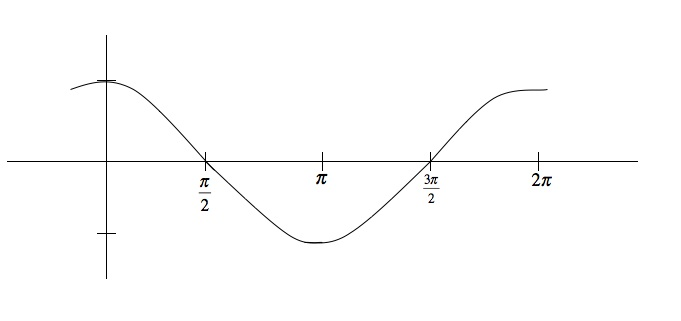• Now graph the amplitude f(x) = 3cos(x)
•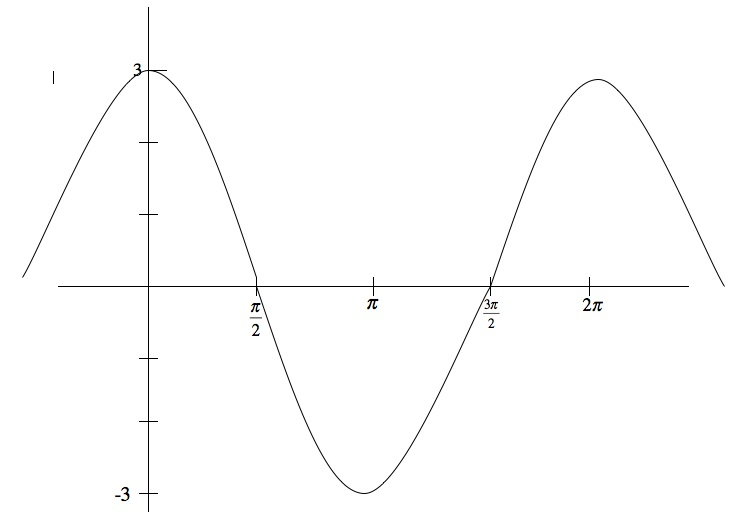• Now graph the period. Since the period is 2π, the graph will stay the same
• Now graph the phase shift f(x) = 3cos(x + π). The graph will shift p units to the left
•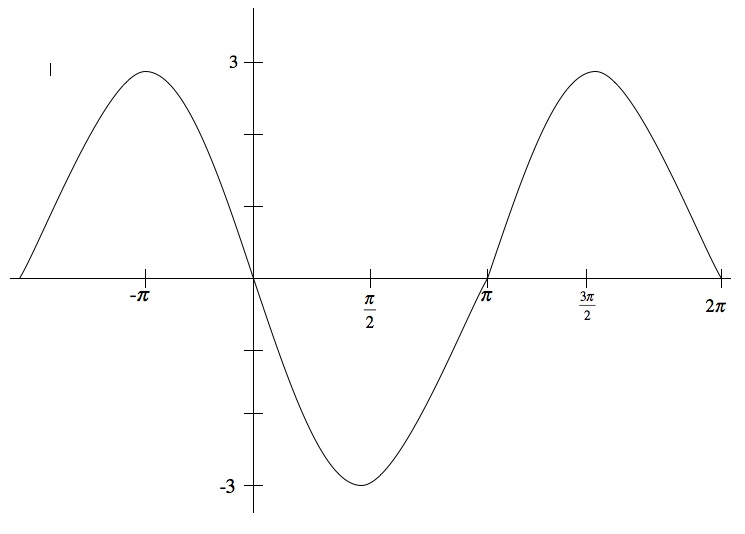• Now graph the vertical shift f(x) = 3cos(x + π) - 3. The graph will shift down from 3 to 0 and - 3 to - 6
•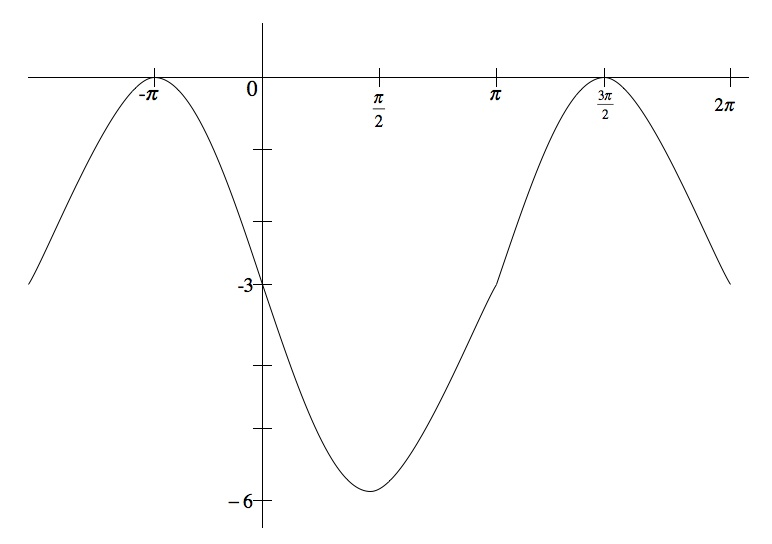Amplitude = 3, Period = 2π, Phase Shift = − π, Vertical Shift = - 3Identify the amplitude, period, phase shift, and vertical shift of the following function. Graph the function.
2sin(2x - [(π)/3]) + 2
• Recall: Asin(Bx + C) + D where |A| is the amplitude; [(2π)/B] is the period; − [C/B] is the phase shift; D is the vertical shift
• Amplitude = |A| = 2, Period = [(2π)/2] = [(2π)/2] = π, Phase Shift = − [C/B] = − [( − [(π)/3])/2] = [(π)/6], Vertival Shift = D = 2
• First graph f(x) = sin(x)
•• Now graph the amplitude f(x) = 2sin(x)
•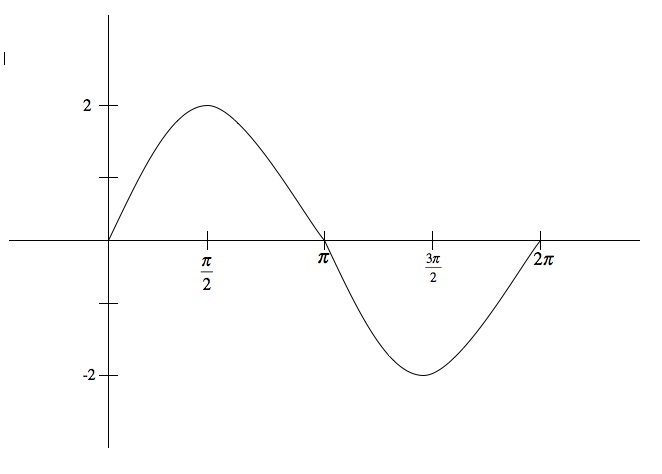• Now graph the period. The period is p, so the graph will cycle every p for f(x) = 2sin(2x)
•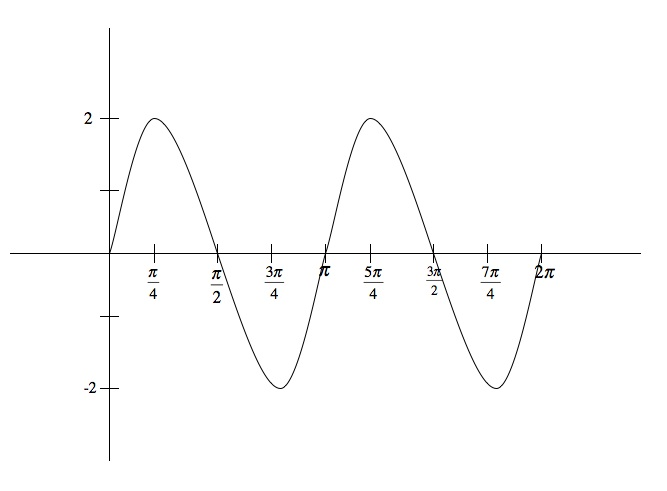• Now graph the phase shift f(x) = 2sin(2x - [(π)/3]). The graph will shift [(π)/6] units to the right
•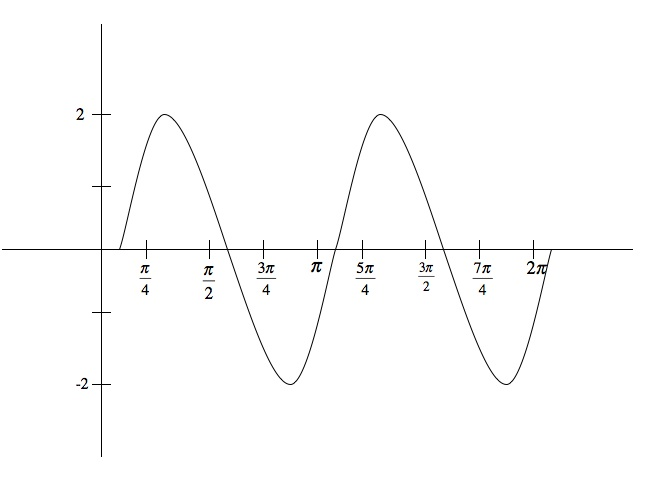• Now graph the vertical shift f(x) = 2sin(2x - [(π)/3]) + 2. The graph will shift up from 2 to 4 and - 2 to 0
•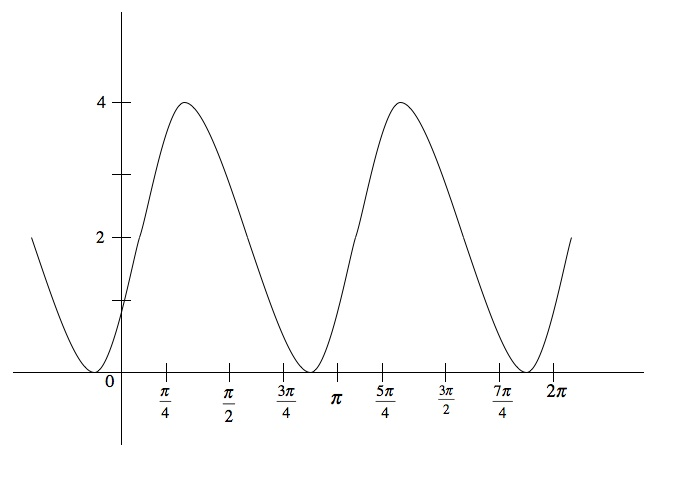Amplitude = 2, Period = π, Phase Shift = [(π)/6], Vertical Shift = 2Identify the amplitude, period, phase shift, and vertical shift of the following function. Graph the function.
- cos([1/2]x − [(π)/4]) + 2
• Recall: Acos(Bx + C) + D where |A| is the amplitude; [(2π)/B] is the period; − [C/B] is the phase shift; D is the vertical shift
• Amplitude = |A| = 1, Period = [(2π)/2] = [(2π)/([1/2])] = 4π, Phase Shift = − [C/B] = − [( − [(π)/4])/([1/2])] = [(π)/2], Vertival Shift = D = 2
• First graph f(x) = cos(x)
•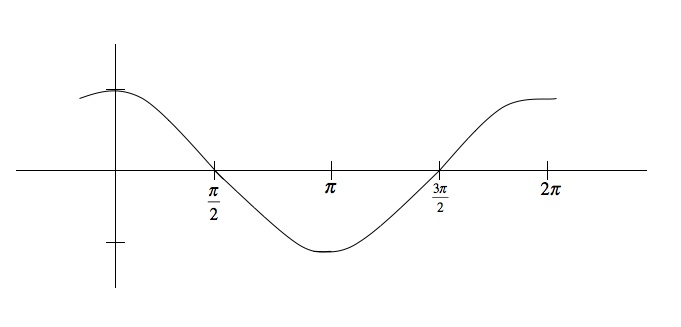• Now graph the amplitude f(x) = - cos(x)
•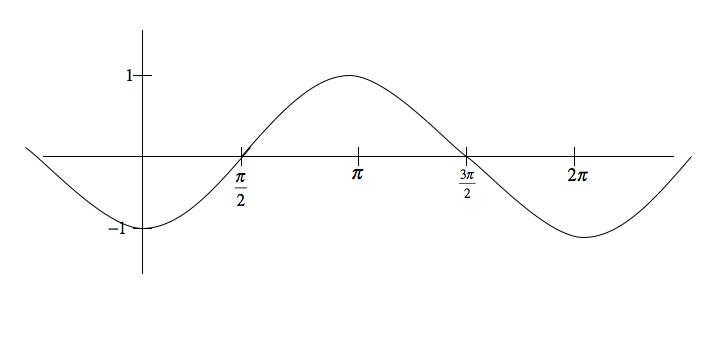• Now graph the period. The period is 4π, so the graph will cycle every 4π for f(x) = - cos([1/2]x)
•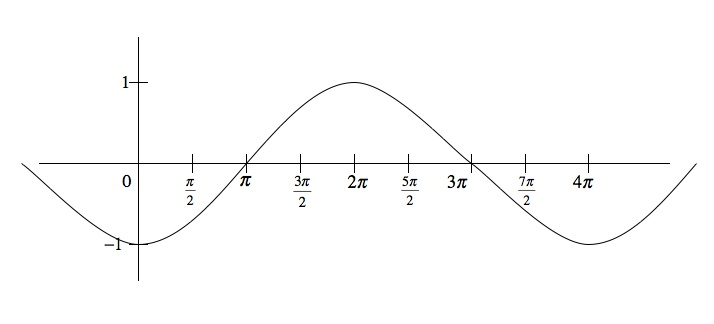• Now graph the phase shift f(x) = - cos([1/2]x - [(π)/4]). The graph will shift [(π)/2] units to the right
•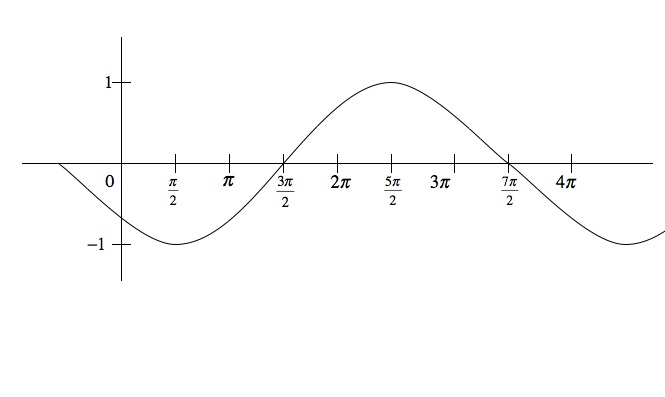• Now graph the vertical shift f(x) = - cos([1/2]x - [(π)/4]) + 2. The graph will shift up from - 1 to 1 and 1 to 3
•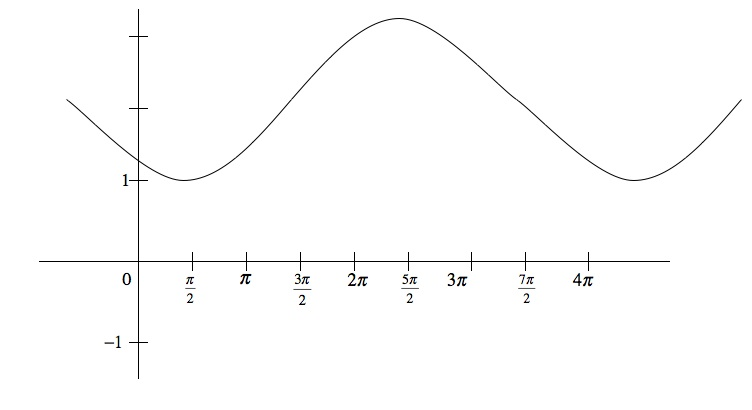Amplitude = 1, Period = 4π, Phase Shift = [(π)/2], Vertical Shift = 2Identify the amplitude, period, phase shift, and vertical shift of the following function. Graph the function.
- 3sin(4x + 4π) - 1
• Recall: Asin(Bx + C) + D where |A| is the amplitude; [(2π)/B] is the period; − [C/B] is the phase shift; D is the vertical shift
• Amplitude = |A| = 3 ,Period = [(2π)/B] = [(2π)/4] = [(π)/2], Phase Shift = − [C/B] = − [(4π)/4] = − π, Vertival Shift = D = - 1
• First graph f(x) = sin(x)
•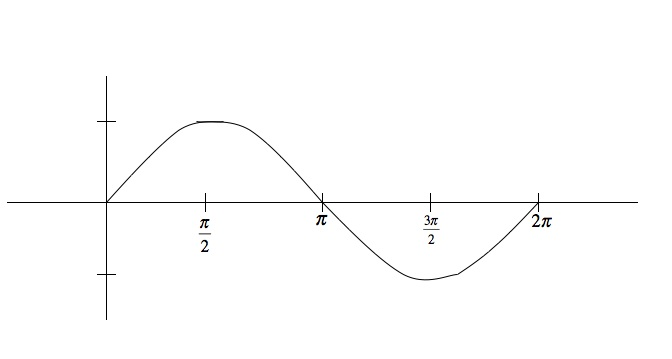• Now graph the amplitude f(x) = - 3sin(x)
•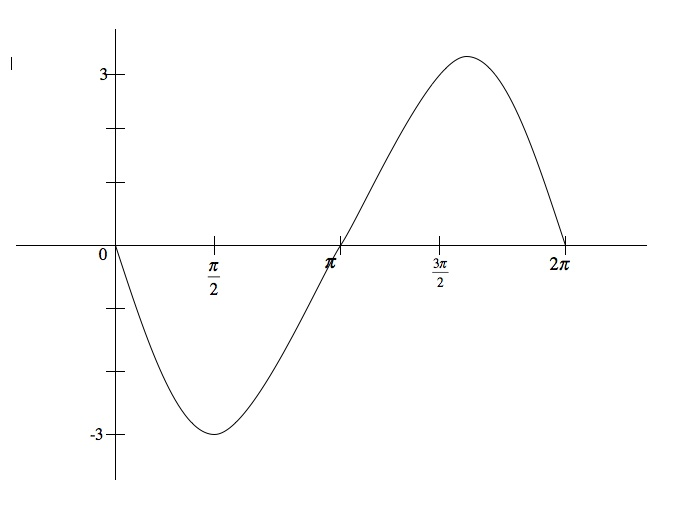• Now graph the period. The period is [(π)/2], so the graph will cycle every [(π)/2] for f(x) = - 3sin(4x)
• Now graph the phase shift f(x) = - 3sin(4x + 4π). The graph will shift π units to the left
•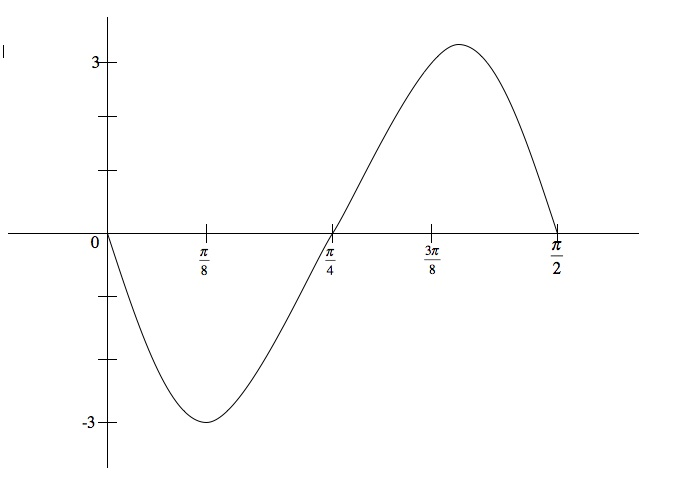• Now graph the vertical shift f(x) = - 3sin(4x + 4π) - 1. The graph will shift down from 3 to 2 and - 3 to - 4
•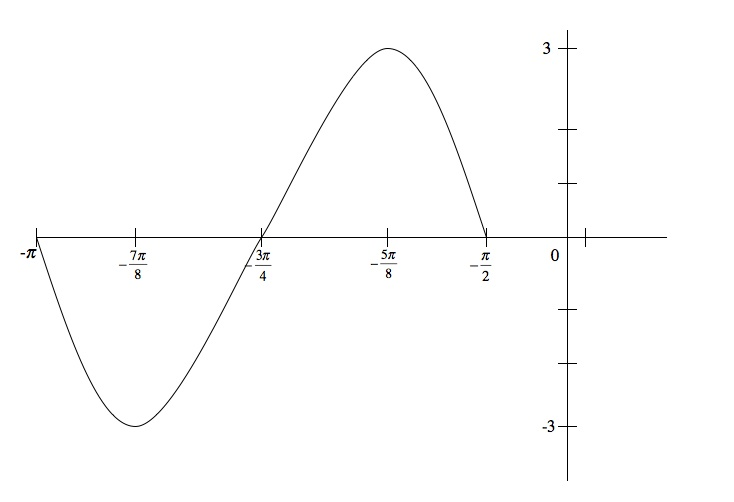Amplitude = 3, Period = [(π)/2], Phase Shift = − π, Vertical Shift = - 1Find a cosine wave with amplitude 4, period [(π)/4], phase shift [(π)/3], and vertical shift of 7
• Recall: Acos(Bx + C) + D where |A| is the amplitude; [(2π)/B] is the period; − [C/B] is the phase shift; D is the vertical shift
• A = 4, D = 7, We need to find B and C by working backwards
• First find B by using the period. We know Period = [(2π)/B] so,
• [(π)/4] = [(2π)/B] ⇒ Bπ = 8π ⇒ B = 8
• Now find C by using the phase shift and the value of B we just calculated
• [(π)/3] = − [C/8] ⇒ 8π = - 3C ⇒ C = − [(8π)/3]
4cos(8x - [(8π)/3]) + 7

*These practice questions are only helpful when you work on them offline on a piece of paper and then use the solution steps function to check your answer.

### Modified Sine Waves: Asin(Bx+C)+D and Acos(Bx+C)+D

Lecture Slides are screen-captured images of important points in the lecture. Students can download and print out these lecture slide images to do practice problems as well as take notes while watching the lecture.

• Intro 0:00
• Amplitude and Period of a Sine Wave 0:38
• Sine Wave Graph
• Amplitude: Distance from Middle to Peak
• Peak: Distance from Peak to Peak
• Phase Shift and Vertical Shift 4:13
• Phase Shift: Distance Shifted Horizontally
• Vertical Shift: Distance Shifted Vertically
• Example 1: Amplitude/Period/Phase and Vertical Shift 8:04
• Example 2: Amplitude/Period/Phase and Vertical Shift 17:39
• Example 3: Find Sine Wave Given Attributes 25:23
• Extra Example 1: Amplitude/Period/Phase and Vertical Shift
• Extra Example 2: Find Cosine Wave Given Attributes

### Transcription: Modified Sine Waves: Asin(Bx+C)+D and Acos(Bx+C)+D

Hi this is www.educator.com and we are going to try more examples of modified sin waves where we start with the basic equation of sin(x) or cos(x) and the graph of sin(x) or cos(x).0000

We introduce these constant which are going to change some of its attributes and we see what that does to the graph.0013

Remember the equation we are working with in general is (a)sin arcos(bx + c) + d and then from each of those values we figured out these various attributes amplitude, period, phase shift and vertical shift.0021

In this particular equation, the amplitude, remember that is just (a) so you read that as 4, the period is 2pi/b , the b is 2 here, so that is 2pi/2 which is pi.0041

The phase shift, that is the strangest one –c/b, that is (–pi/2)/2 or –pi/4.0066

Finally the vertical shift, that is the easier one is -1 here.0085

Again we will start out with the basic sin way, we will work through introducing these attributes one at a time and see how that moves around and create a new function for us.0097

Let us start out with the basic sin way.0108

There is pi, 2pi, so I’m just going to graph sin(x) to start with.0119

I’m going to graph it flat because I’m looking ahead and noticing that the next step is to increase the amplitude to 4.0130

It is really going to be stretched up, I’m going to keep my scale narrow here.0139

The next step is to introduce the amplitude, we are going to graph 4sin(x).0148

That stretches it up and down by a factor of 4, -4 goes down to -4 goes up to +4, that makes it a lot steeper.0156

That is 4sin(x), we got the amplitude incorporated there, remember it is very important to do these in order, amplitude, period, phase shift, vertical shift.0175

Next step is to introduce the period, right now the period is 2pi and we want the period to be pi.0185

Instead of doing a full cycle in 2 pi units, that is going to do cycle in pi units, that means it shuffles twice as fast.0197

Let me try and draw that.0205

That is pi/2 there, 3pi/2, ok we got the period incorporated.0220

What I really graphed there was 4sin(2x), next we want to incorporate the phase shift.0230

I will be graphing 4sin(2x) + pi/2 and this is starting to get a little crowded here, so I’m going to do this one in red.0241

That means that the phase shift is –pi/4, that means we move –pi/4 units to the left.0257

Instead of starting at 0, I’m going to start at –pi/4 and it came back down before pi/2 so now it is going to come back at pi/4.0267

It used to come back up at pi and now it comes back up at 3pi/4.0287

That red graph is what we get by incorporating the phase shift and finally we will incorporate the vertical shift and I will do this one on blue.0301

We are going to be doing 4sin(2x + pi/2) -1, that means we take the graph and we move it down by one unit.0316

It is not the same as changing the amplitude where things stretch up and down.0328

Here we are not stretching anything, we are just moving everything directly down by one unit.0331

Instead of starting at 0 going up to 4 down to -4, everything is moved down by 1 unit.0336

It will peak at three and instead of going down to -4, it will go down to -5 and the middle part will be at -1.0346

Let me go and try to graph that in blue.0355

It comes back to -1 instead of 0, bottoms out at -5 instead of -4, goes back up peaks at three, back down to -5 and back up to -1 again.0362

This blue curve is the final graph there, it is very complicated by the time it is all over but it is a bunch of simple steps.0382

The first step is to look at the equation and identify these quantities, amplitude, period, phase shift, and vertical shift.0395

We got pretty simple equations for each one of those, the tricky part of the graphing is where you start with sin(x).0403

You remember how to graph sin(x), that is very easy and then start incorporating these attributes one by one.0414

Amplitude stretches it vertically up and down, period stretches or compresses it horizontally, in this case the period was pi and since it was 2pi/4 we have to compress it by a factor of 2.0420

Phase shift moves it over to left and right, vertical shift moves it up or down.0434

We finally end up with this blue curve that has been modified according to all those attributes.0440

Now we are being asked to find a cos wave and we are given the attributes but we are not given the equations.0000

We have to reverse engineer the equation from this attributes.0007

Remember the equation we are going to go for is (a)cos(bx+c)+d, but we do not know what a, b, c, and d are, we got to figure them out from these attributes.0012

Amplitude is the opposite value of a, we will take a=2 to give ourselves amplitude =2.0028

The period is 2pi/b and that is supposed to be equal to 3pi.0048

From that we can figure out if we solve that for b, we get (b) is equal to 2/3, that is how we can figure out what (b) should be.0063

The phase shift is –c/b which is supposed to be equal to pi/2, now that requires a little bit of solving, so we get –c/b we already figure out that was 2/3 is equal to pi/2.0073

That is -3(c)/2 is equal to pi/2, the 2 is cancel and so we get (c) is equal to –pi/3.0106

That is how we figured out what (c) is, we reversed engineer this equation –c/b.0122

Finally the vertical shift, is (d) which is -2, we figured out what a, b, c, and d are, that means that our equation is a=2cos(2/3x)-(pi/3-2).0128

We figured out the first part of the problem which is to find the equation, now we are going to do the tricky part which is graphing it.0162

It is not so tricky if you start with the basic cos wave if you remember how to graph that and then you introduce this attributes in the right order.0168

I will start with the basic cos wave, cos(x), there is pi, 2pi, 3pi. Cos(x) I know it starts at 1, there is 1, -1, it starts at 1, it goes down to 0.0179

Bottoms out at -1 goes back up to 0, goes back up to 1, now I got cos(x) that I pretty much do from memory.0217

Next I’m going to do is introduce the amplitude, I’m going to talk about 2cos(x) that just stretches it vertically up and down, it goes up to 2 and down to -2.0228

Next I’m going to adjust the period, what I’m actually going to be graphing is 2cos(2/3x).0257

I know that makes the period 3pi, our original period is 2pi, this is stretching it out by a factor of 50% instead of doing a full cycle in 2pi, it is going to do a full cycle in 3pi.0269

Let me give my self some coordinates here, it still going to start at 0 but it is going to bottom out now.0286

It is going to finish up at 3pi instead at 2pi, it is going to bottom out halfway between them that is 3pi/2.0295

I took the graph and I stretched it out so that it has a period of 3pi now, phase shift –pi/3.0324

What I’m going to be graphing is 2cos(2/3x)-pi/3, sorry the phase shift is pi/2, the c value is –pi/3 but the phase shift is pi/2.0338

I’m going to take this graph and move it over pi/2 units to the right.0358

It looks like it is getting a little complicated now, I’m going to switch into red here.0364

We are going to graph the phase shift in red so that means instead of starting at 0, I’m going to start at pi/2.0371

It is going to bottom out at 2pi and it is going to come back up at 3pi + pi/2.0379

My orientation points were pi/2, 2pi, 3pi + pi/2, because I just took my orientation points before and move them all over by pi/2.0413

That incorporated the phase shift of pi/2, we are almost done here.0425

We got on more step to incorporate which is this vertical shift of -2.0430

I’m going to do this one in blue so we are going to be graphing 2cos(2/3x)-(pi/3-2) that means we are going to take the whole graph and just move it down 2 units.0435

Now remember, before our peaks and values were 2 and -2, we moved that down by 2 units and the peaks and values are now going to be at 0 and -4.0455

I’m going to extend my axis down to -4, -3, -4.0467

I’m going to take my reference points from before and move them down by 2 units.0476

This graph peaks at 0 and it never actually goes north of the x axis because it is going up to 0 and down to -4.0505

That is the final graph that we have been asked for, let us recap there.0520

We are given all these attributes amplitude, period, phase shift, and vertical shift.0528

We know the equation for those 4 things, absolute value of a, 2pi/b, -c/b and d.0533

What we do is we plug in those attributes and reverse engineer to figure out what a, b, c, and d are.0541

Then we can put those together to get the equation of the function that we are trying to graph.0550

To actually graph it, we start with basic cos curve, we introduced the amplitude.0557

It is very important to do this in order, introduce the amplitude where you expanded it up, up and down.0563

The thing actually stretches out, we introduced the period which collapses it horizontally or stretches it out horizontally.0570

In this case, we are changing the period from 2pi to 3pi, so that stretches it horizontally.0581

We introduced the phase shift which moves it horizontally to the right or left without stretching it.0587

The period was the part where you stretch it, the phase shift just moves it without stretching it.0593

Finally, we introduced the vertical shift which moves it up and down without stretching it, in this case it moved down.0599

At the end of it, it is quite a complicated process but individually each one of these steps if you keep them in order is not to hard.0608

You just start with you original cos graph and then move them around according to these steps until you get the one you want.0615

That is the end of our lecture on modified sin waves, this is www.educator.com.0622

Hi this is educator.com and today we're going to learn about modified sine waves.0000

We're going to learn how to analyze and graph these functions given by, instead of just talking about sin(x) and cos(x), now we're going throw a whole bunch of constants in there.0007

We'll talk about asin(bx+c) and then throw a constant outside +d and the same kind of thing with cosine.0020

That takes a basic sine or cosine graph and it moves it all around.0027

We're going to learn some vocabulary to describe those movements and we're going to learn how to graph those.0030

First of all, some vocabulary, remember we're talking about the graph asin(bx+c)+d.0038

We're talking about sine waves, these are functions that basically have this shape like sin(x), but they maybe moved in different waves.0057

We need some vocabulary to describe the different ways that could be moved.0073

The first one that we're going to learn is amplitude.0079

The amplitude of the sine waves is the vertical distance from the middle of the waves to the peaks.0082

What that represents graphically is this distance right here, that's the amplitude.0089

Of course, that's the same as this distance, that's also the amplitude but you can measure it either way.0102

If the wave is moved up, if it's floating up above, the x axis, somewhere like that, then the amplitude is still the distance from the middle of the wave to the peaks.0114

In terms of equations, it's very easy to spot the amplitude.0129

When you're given asin(bx+c)+d, the amplitude is just that number a, or if the a is negative, you just take the absolute value.0133

It's just the positive version of that number a that tells you the amplitude right there.0148

It tells you how far up away it's going to the peaks, how far down it's going to the valleys.0158

The period of a sine waves, I'll show this in red, is the horizontal distance for the wave to do one complete cycle from one peak to the next peak.0160

That is the period right there, of that wave, that's the period.0179

On that one, that's the period right there.0189

Remember, when you're working out the equations, remember that if you have sin(x) that has period 2π, it takes 2π to repeat itself.0198

We learned that when we looked into the original sine graphs.0212

If you have sin(2x), that makes it wobble up and down twice as fast, the period would be πsin(4x), the period would be π/2.0215

The pattern that you noticed here is it that the period is given by 2π over the coefficient of x, 2π/b.0230

The b there tells you where the period is, not the b itself but you plug b into that equation and that tells what the period is.0242

Two more vocabulary words we need to learn, the phase-shift of a sine wave is the horizontal distance that the wave is shifted from the traditional starting position.0254

Let me rewrite the equation here, asin(bx+c)+d.0269

The traditional starting position for sine would be at (0,0), and the traditional starting position for cosine would be at (0,1).0280

Those are the traditional starting positions but the phase-shift will move the graph to the right or the left.0302

In these equations, it's given by -c/b, and that seems a little mysterious and let me explain that a little bit.0311

We can write this bx+c, first of all, we can factor b out, we can write that b[x + (c/b)].0320

Then, we can write that as b[x - (-c/b)].0332

That's where that -c/b comes from.0340

If you have trouble remembering that formula -c/b, you can go through this little process to remember that, x-(c/b), that shows you that it moves it c/b units to the right.0342

Let me draw that, I'll draw that in blue.0367

If you're starting with a sine curve, the phase-shift is the amount that it moves over, that's the phase-shift right there, it moves it over -c/b units.0370

If you're starting with a cosine curve, that's that phase-shift right there.0393

Finally, the vertical shift is what happens when you take the graph and you just move it up or down vertically without changing anything else.0407

If we apply a vertical shift to that, that amount right there, I'll draw this in red, that's the vertical shift.0434

That's a little bit easier to pick out than some of the others, because that's just the d in the original equation.0455

If you're moving the graph up or down by an amount of d.0464

This can get pretty tricky we're starting with the basic sine and cosine curves, but then we're moving around and stretching them out, and moving them up and down in all different ways.0469

It's a little bit tricky but we'll go through some of the examples and you'll get the hang of it.0479

In our first example here, we're given an equation 3cos(4x+π)+2.0485

We have to identify all these various things, the amplitude, the period, the phase-shift and the vertical shift and then we're going to draw a graph of the function.0495

The key thing here is it if you identify these things in order, then it becomes very easy to pick them out using the equations.0502

The graph isn't too hard as long as you these things in order.0510

The amplitude, remember, that's just the number on the outside, the a in the original equation.0517

Let me write down the original equation, acos(bx+c)+d.0528

The amplitude is just the a right there, that's the 3.0539

The period is 2π/b, the b there is 4, that's 2π/4 which is π/2.0543

The phase-shift is -c/b, our c here is π, -π, b is 4, that's -π/4.0563

The vertical shift is just that last term d, which is 2.0580

Those are the answers to the first part of the question.0596

Trickier part is doing the graph and there's a general strategy for doing this graphs that sort of always works, but you really have to follow it closely.0602

The strategy is to start with the basic cosine graph, which hopefully you remember how to do it, you start with the basic cosine graph.0611

Then move it around according to each one of these parameters.0620

The key thing here is you have to do it in order, you have to do amplitude period phase-shift then vertical shift.0624

Let's see how that works out.0630

Let me draw a basic cosine graph and then we'll try moving it around according to these different parameters.0634

Remember, basic cosine graph, there's π, there's 2π, π/2, 3π/2.0645

Basic cosine graph starts at 1, goes down to 0 at π/2, -1 at π, up to 0 at 3π/2, and it's 1 again at 2π.0661

That's the basic cosine graph, you pretty much have to remember that to get started here.0676

First thing we're going to do, is we're going to change the amplitude.0682

Let me keep track of this as I go along.0688

First one we graphed was cos(x), just y=cos(x).0692

Next we're going to graph is 3cos(x), we're going to bring in the amplitude.0697

What that does is that it stretches up the peak, then it stretches down the valleys by a factor of 3.0700

I'm going to draw the same shift graph but three times as tall, and three times as deep.0709

Instead of going from 1 to -1, goes down to -3 and up to 3.0720

That second graph I drew there was 3cos(x).0725

The next one is to introduce is the period.0734

The period is supposed to be π/2, remember, the period is the amount of horizontal distance between one peak and the next peak.0738

My current peak is...0747

I'm going to change the period to π/2.0754

What I'm really graphing here is 3cos4x.0760

What that's going to do is, instead of having a period 2π, it shrinks it horizontally, or it compresses it horizontally so that it does a complete period in the space of π/2.0769

There's π/2 right there.0784

I need to do a whole period between there and there.0787

Every π/2, it does a complete cycle.0800

What I just drew was 3cos4x.0811

The phase-shift I'm going to introduce is -π/4, that means it moves it π/4 to the left and it's getting a little bit messy.0817

I'm going to see if I can draw this in red or will see if it's still visible.0827

Instead of going from 0 to π/2, I'm going to draw my graph from -π/4 to π/4, because we're moving it to the left by π/4.0832

That's the graph in red there, 3cos4x+π because I've introduced the phase-shift in there.0852

Now, it's really going to get messy if I try to draw any more on the same axis, so I'm going to set up a new set of axis.0860

I'll redraw the red one on this set of axis, -π/2, and that one is going from 3 to -3.0883

Remember the red one is the previous graph shifted over by -π/4.0911

Finally, we need to introduce the vertical shift of 2, and I'll do this last graph in blue.0916

That takes the entire graph and raises it up by 2 units.0922

That means instead of going to 3 from -3, it's going to go up in π, and instead of going down to -3, it only goes down to -1.0927

Let me label that more clearly, -1.0942

I'll draw this one in blue, this is now 3cos4x+π, and I'm introducing the vertical shift of +2.0947

I'm taking the graph and I'm moving it up 2 units there.0965

That blue graph at the end is our final function.0972

This is really a pretty complicated process.0980

There's a lot of steps involved but each individual step is not that hard, and if you do them in order and you're careful about each one, it's not too bad.0983

Let me just recap there, we started with the original graph of cos(x), that's the starting point.0993

Then we introduced the amplitude, and that stretches it vertically by a factor of 3, stretches it up and down.1000

We introduced the period which compresses it horizontally.1013

We introduced the phase-shift, which takes the whole thing and it moves it to the right or the left.1020

Finally, We introduced the vertical shift which takes the whole thing and moves it up or down.1030

Remember, it's important to do these in order, amplitude, period, phase-shift, vertical shift.1041

If you do those out of order, then they'll mess each other up as you go along.1050

We really want to do those in order.1055

We want to practice several of these, so let's get moving in other example.1059

Here's another example, same questions here, amplitude, period, phase-shift, and vertical shift of the following function.1061

Remember we can read this off quickly, just remembering the formula asin(bx+c)+d.1070

If we can figure out what a, b, c, and d are, we have formulas for all of these properties.1080

Amplitude, that's the a, or if the a is negative, make it positive, that's the absolute value of a, which is just 2 here.1089

The period is 2π/b, the b is 2 here, so that's π.1098

The phase-shift is -c/b, which is, okay, c is -π/3, so negative of that is (π/3)/2 will give us π/6.1109

Finally, the vertical shift is d, which ,in this case, is just 0.1135

Finally, the fun part, we get to graph the function1154

Remember, you always start with your basic sine or cosine graph and then you start moving it around according to these different parameters but you got to keep these parameters in order.1159

I know the basic shape of the sine graph, I've got that memorized, π and 2π.1174

I know sine always starts at 0, goes up to 1, comes back down to 0, to -1, and then back to 0.1187

There's 1, -1, there's π, π/2, 3π/2, so that's my basic sine graph.1199

That's the first thing I graphed there, sin(x), remember this is the graph of sine and cosine x.1209

Now, we start introducing these other attributes and it's important to go in order.1217

First of all, we're going to introduce the amplitude.1222

The amplitude is 2, but we're really multiplying the graph by -2, -2sin(x) is what I'm going to graph next.1225

-2sin(x) that stretches it vertically because of the 2, but it also flips it vertically.1235

Instead of starting by going up, it's going to go down, goes down to -2, up to 0, up to 2, and back down to 0.1246

That's first one was a little lop-sided, let me just see if I can make that a little more, a little smoother.1270

Okay, we've got -2sin(x), look it's got a bigger amplitude than the original graph and it's flipped over because of the negative sign.1280

Next thing I'll introduce is the period.1288

The period is supposed to be π, so I'm graphing -2sin(2x).1292

That speeds the whole thing up, it shortens the period because the period is now π instead of 2π.1298

I need to do that entire graph in the space of π instead of 2π.1306

There, that one that I just graphed, I shortened the period to be π instead of 2π.1330

The period is now π on that new graph.1337

Next, we're going to do the phase-shift, that's π/6 units to the right.1340

The phase-shift, what I'm about to graph is -2sin(2x)-π/3, so that takes the whole graph and it shifted over π/3 units to the right.1349

Let me do this one in red.1364

I'm going to take that last graph and shift it over π/3 units to the right.1370

Instead of starting at (0,0), it starts at π/3, it's going to come back down to 0, at 5π/6.1374

The phase-shift is supposed to be π/6, so I'm going to move everything over by π/6.1389

Instead of starting at 0, I'm going to start at π/6 and come back at (π/2)+(π/6) which is actually 2π/3.1408

That red curve that I graphed there is -2sin(2x)-π/3.1439

The last step is to do the vertical shift which is 0, so we don't have to move the graph at all which means we're done.1453

This last graph is the one we want.1464

Again, it's a matter of breaking these equations down into their parts.1470

It's very complicated if you kind of look at the whole thing but if you look at each steps and you keep each steps in order then it's not to hard.1475

Remember the equations for amplitude, period, phase-shift, and vertical shift.1484

Once you've got those, you start with your basic sin(x) graph then you change the amplitude which stretches it out up and down, or might flip it.1489

You change the period which compreseses it horizontally.1500

You do the phase-shift which takes the whole thing and without compressing it, it moves it to the right or the left.1507

Finally, the vertical shift moves it up or down.1511

You could just keep moving these graphs around until you build up the equation you're looking for.1515

This one's a little bit different, we're asked to find the sine wave.1526

This time we're told what all the properties are.1530

We're given the amplitude, the period, the phase-shift, and the vertical shift.1535

We want to find an equation and we want to graph the function.1540

I'm going to kind of build this up, the same way we're building the earlier graphs.1543

I'm going to start with the basic sin(x), so that's my basic sine wave.1548

Now, I'm going to give an amplitude 2, and remember 2 is just a number on the outside, the a.1554

Let me rewrite that equation, asin(bx+c)+d.1559

Amplitude 2 means a is 2, 2sin(x).1566

Now, period 4π, remember our equation for period was 2π/b, that is supposed to be equal to 4π.1575

When you solve that out, that tells us that b=1/2, so the b has to be equal to 1/2.1597

That means our equation is now 2sin(1/2)x, so we've incorporated the period.1610

Phase-shift is supposed to be π/2, but remember the phase-shift, our formula for that is -c/b.1622

b is already, we figured out as 1/2, so that's -c/(1/2).1643

If we do a little bit of algebra here, we get (1/2)π=2c, so c=-π/4.1650

I got that from the equation -c/b=π/2.1667

I already figured out my b, now I can figure out my c.1672

The next part of that equation is 2sin[(-1/2)x-(π/4)].1677

Finally, I want to talk about the vertical shift, which is supposed to be 1 and that's the d.1692

Finally, my equation is 2sin[(1/2)x-(π/4)]+1.1704

That is the sine wave that I'm looking for.1715

Now, I want to graph that thing.1722

I start out with the basic sine wave, π, 2π, π/2, 3π/2.1730

Basic sine wave starts at 0, goes up to 1, back down to 0 at π, down to -1, back up to 0 at 2π, basic sine wave.1742

Now, I'll introduce these properties in order.1753

I'll start out with the amplitude.1755

Amplitude's supposed to be 2, so I'm going to stretch this thing up instead of going from 1 to -1, it's going to go up from 2 up to 2, and down to -2.1760

It'll stretch the thing up, and down.1774

Period is 4π, that means the thing stretches out, so that it'll only does one cycle every 4π.1783

That means I have to extend my graph quite a bit here, 3π, 4π, so I'll stretch the thing out, so it'll only does the cycle every 4π.1794

Now, phase-shift π/2, that means the thing is going to shift π/2 units to the right.1817

I better draw a new set of axis here.1824

I've got π, 2π, 3π and 4π, 5π.1835

What I want to do is take the graph I have above and move it π/2 units to the right because we have phase-shift π/2.1850

Instead of starting at 0, I'm going to start at π/2, I go up to there, back down to 0 at 2π plus π/2, back down to -2 between 3 and 4π and back up to 0 there.1866

So, connect these up.1887

Finally, I have to do one with vertical shift 1, that means I'll take the whole graph and I'll move it up by one unit.1913

That means instead of going or peak at 2, it's going to peak at 3 now.1920

Instead of going down to -2, it gets moved up by 1 unit so it's going to go down to -1.1932

Let me draw this last final curve in red.1940

Everything gets moved up by 1 unit.1948

I'm going to plot some points here, moving everything up by 1 unit.1953

That final curve is the one we want.1976

That's 2sin[(1/2)x-(π/4)]+1.1980

Again, it's a complicated procedure but if you take it step by step, each one of the steps is not too hard.1991

First, we kind of reconstructed the equation from these parameters that we were given.1997

Basically we figured out a, b, c and d from these parameters that we were given by sort of reverse engineering the formulas 2π/b, -c/b, and the d.2004

Then, we went through step by step.2020

We started with the basic sine curve.2024

We changed its amplitude, stretched it vertically.2030

We changed its period which stretches out horizontally.2034

We changed its phase shift which moved it over horizontally not stretching but just moving it without stretching.2036

Then, we did the vertical shift, moving it up or down.2041

You should practice a few of these curves on your own.2045

We'll come back and try some more examples together later.2047

OR

### Start Learning Now

Our free lessons will get you started (Adobe Flash® required).